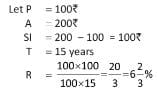# Test: Simple Interest & Compound Interest- 1

## 19 Questions MCQ Test UPSC Prelims Paper 2 CSAT - Quant, Verbal & Decision Making | Test: Simple Interest & Compound Interest- 1

Description
Attempt Test: Simple Interest & Compound Interest- 1 | 19 questions in 30 minutes | Mock test for Quant preparation | Free important questions MCQ to study UPSC Prelims Paper 2 CSAT - Quant, Verbal & Decision Making for Quant Exam | Download free PDF with solutions
QUESTION: 1

### At the rate of 6% p.a. simple interest, a sum of Rs. 2500 will earn how much interest by the end of 5 years.

Solution:

Simple interest = ( P x R x T) / 100

= (2500 x 6 x 5) / 100

Simple interest = Rs. 750

QUESTION: 2

Solution:
QUESTION: 3

### In what time will Rs. 72 become Rs. 81 at 25/4% per annum simple interest?​

Solution:

Interest = Rs. (81-72) = Rs. 9
Let the time be t years.
9 = (72×25×t)/(4×100)
t = (9×400)/(72×25)=2 years

QUESTION: 4

At what rate percent per annum will a sum of money double itself in 15 years.

Solution:QUESTION: 5

In what time Rs. 2600 amount to Rs. 3055 at the rate of 3 ½ % per annum.

Solution:

The correct option is B.
Principal amount=2600
Rate=3 1/2%pa=7/2
Time = T
SI=3055-2600=455
SI=PRT/100
455=2600×7×T/100×2,
T=5yrs.

QUESTION: 6

Find the sum of money when increases 1/10 of itself every year amount to Rs. 600 in 5 years.

Solution:
QUESTION: 7

A sum of money amounts to Rs. 1100 in 2 years and Rs. 1400 in 6 years at simple Interest. Find the rate percent.

Solution:
QUESTION: 8

In what time Rs. 540 at 5 percent per annum will produce the same Interest as Rs. 1800 in 5 years at 6 percent per annum.

Solution:
QUESTION: 9

The simple Interest on a certain sum of money at the rate of 4% p.a. for 5 years is Rs. 1680. At what rate of interest the same amount of interest can be received on the same sum after 4 years ?

Solution:

5%
S.I. = 1680, R = 4% T = 5 years
Principal = (100 *1680)/ (5*4) = 8400
So P = 8400
Rate = (100 *1680)/ (8400 * 4) = 5%

QUESTION: 10

A lent 180 to B for 10 years and 200 to C for 2 years at Simple interest, the rate of interest being same in both the cases. He received Rs. 220 as total interest. Find the rate%.

Solution:
QUESTION: 11

A man had Rs. 3000 a part of which he lent at 4% andrest a 5%. The whole annual interest received was Rs. 130. The money he lent at 4% was ……?

Solution:
QUESTION: 12

A man had Rs. 5800 a part of which he lent at 4% and rest at 6%. The whole annual interest received was Rs. 292. The money lent at 4% was ……?

Solution:

The correct option is D as Let the part lent at 4% be x then , rest will be (5800-x) .
(x*4*1)/100 + { (5800-x)*6*1 } / 100 = 292
4x/100 + { 34800 - 6x }/100 = 292
4x + 34800 - 6x = 29200
2x = 5600
x = 2800 so , d is correct option.

QUESTION: 13

A man buys a land and gives for it 20 times the annual rent. Find the rate of Interest he gets for his money.

Solution:

Let annual rent is 1 Rs. so buys the land at 20 Rs. So by investing Rs.20 he is getting Rs.1 as interest. so on Rs.100 he gets Rs.5. So rate%=5%. Hence option D is the answer.

QUESTION: 14

At what rate percent per annum simple Interestwill a sum of money be 1.1 times in 2 years?

Solution:

The correct answer is D as the rate would be 5 % which is not in given options . so the D  would be the correct option.

QUESTION: 15

Find the Compound Interest on Rs. 12500 at 8% per annum for 9 months compounded quarterly.

Solution:

The correct is D as
n=9 months
=3 quaterly
A=125000×(1+2/100)3^=125000×(1+2/100)^3
=125000×51/50×51/50×51/50=125000×51/50×51/50×51/50
=Rs.132651
CI=A−P
=132651−125000
=Rs.765.1

QUESTION: 16

Find the rate of Simple Interest when a sum of money treble itself in 25 years.

Solution:
QUESTION: 17

Adam borrowed some money at the rate of 6% p.a. for the first two years, at the rate of 9% p.a. for the next three years and at the rate of 14% p.a.for the period beyond five years.1£ he pays a total interest of Rs. 11, 400 at the end of nine years how much money did he borrow?

Solution:

Let the sum borrowed be x.

Then,(x*2*6)/100 + (x*9*3)/100 + (x*14*4)/100 = 11400

⇒ (3x/25 + 27x/100 + 14x / 25) = 11400

⇒  95x/100 = 11400

⇒  x = (11400*100)/95 = 12000.

Hence , sum borrowed = Rs.12,000.

QUESTION: 18

The Simple Interest on a certain sum of money for two years is Rs. 40 and the CI on same sum at same rate for same time is Rs. 40.50. Find the sum.

Solution:
QUESTION: 19

Invested a sum of money at CI. It amounted to Rs. 2420 in 2 years and 2662 in 3 years. The rate percent is …….

Solution: Diff.in amount=
=2662-2420=242
:-242 is the interest on on2420 in one year( it is same for SI or CI because both are same for one year)
hence rate of interest= 242×100/2420=10%Use Code STAYHOME200 and get INR 200 additional OFF Use Coupon Code